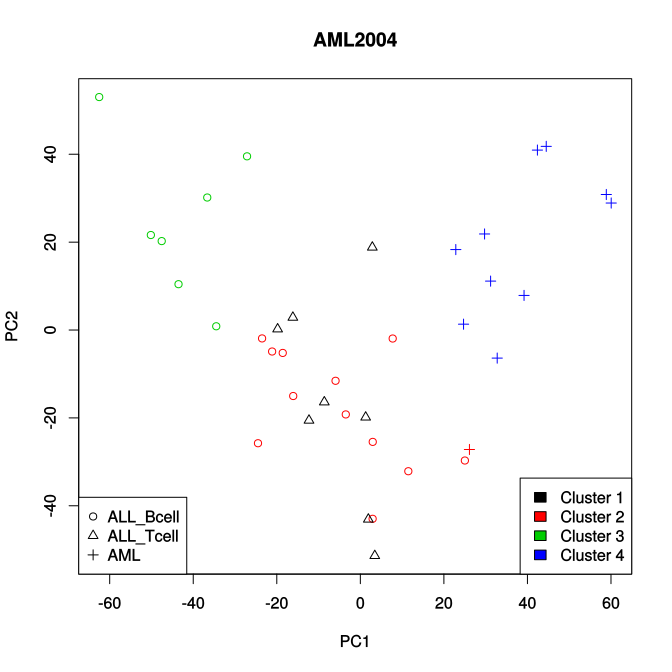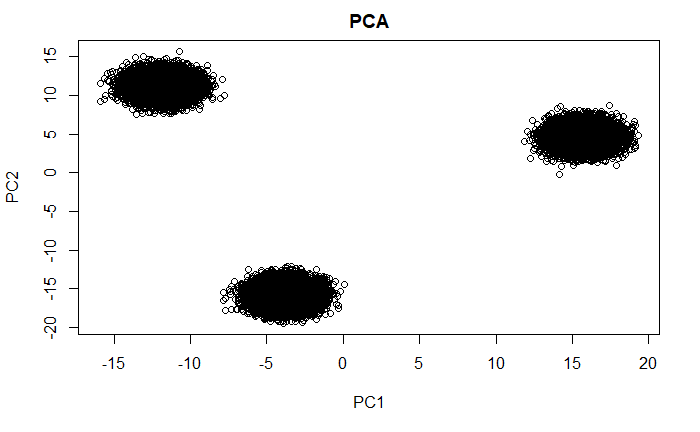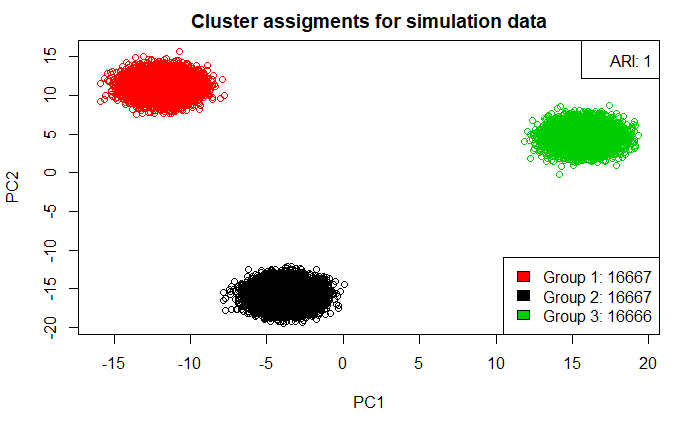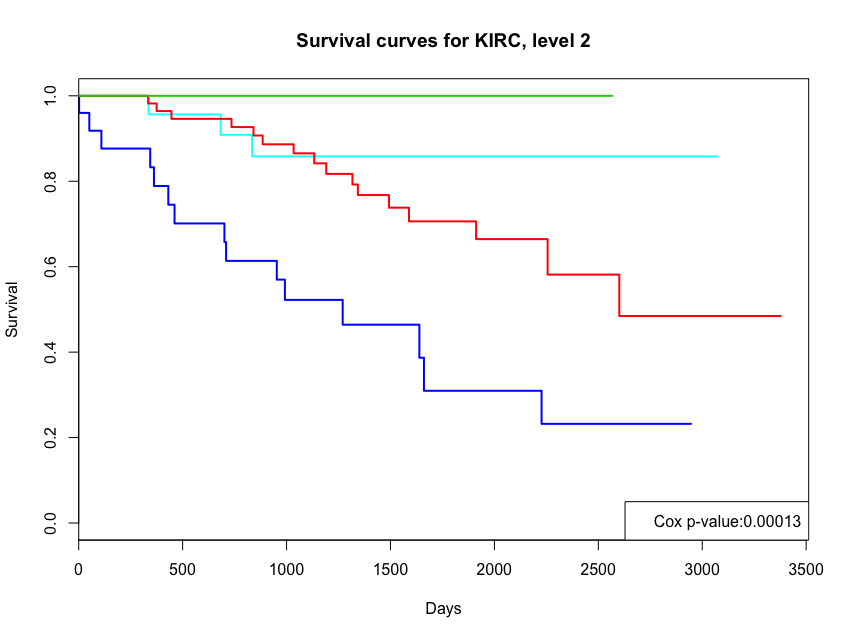\tableofcontents

\newpage

\section{Introduction} In recent articles published in Genome Research [@nguyen2017novel] and Bioinformatics [@nguyen2019pinsplus], Nguyen et al. proposed a perturbation clustering approach for multi-omics data integration and disease subtyping called PINS. The framework was tested upon many datasets obtained from The Cancer Genome Atlas (TCGA), the European Genome-Phenome Archive and simulation study. Please consult Nguyen et al. [@nguyen2019pinsplus;@nguyen2017novel; @nguyen2017horizontal] for the mathematical description.

PINS+ offers many improvements of PINS from practical perspectives. One outstanding feature is that the package is extremely fast and highly scalable. For example, it takes PINS+ only two minutes using a single core to analyze the Breast invasive carcinoma (BRCA) dataset (622 patients with three data types, mRNA, miRNA, and methylation) while it takes PINS 236 minutes (almost four hours) to analyze the same dataset. For more details on big data analysis, please consult Nguyen et al. [@nguyen2021smrt].

This document provides a tutorial on how to use the PINS+ package. PINS+ is designed to be convenient for users and uses two main functions: PerturbationClustering and SubtypingOmicsData. PerturbationClustering allows users to cluster a single data type while SubtypingOmicsData allows users to cluster multiple types of data.

\section{PerturbationClustering} The PerturbationClustering function automatically determines the optimal number of clusters and the membership of each item (patient or sample) from a single data type in an \textbf{unsupervised analysis}.

#### Preparing data

The input of the function PerturbationClustering is a numerical matrix or data frame in which the rows represent items while the columns represent features.

Load example data AML2004

library(PINSPlus)
data(AML2004)
data <- as.matrix(AML2004$Gene)  #### Run PerturbationClustering Run PerturbationClustering with default parameters system.time(result <- PerturbationClustering(data = data, verbose = FALSE))  ## user system elapsed ## 4.264 6.072 0.789  PerturbationClustering supports parallel computing using the ncore parameter (default ncore = 1): result <- PerturbationClustering(data = data)  Print out the number of clusters: result$k

##  4


Print out the cluster membership:

result$cluster  ## ALL_Bcell_1 ALL_Bcell_2 ALL_Bcell_3 ALL_Bcell_4 ALL_Bcell_5 ## 2 2 3 2 2 ## ALL_Bcell_6 ALL_Bcell_7 ALL_Bcell_8 ALL_Bcell_9 ALL_Bcell_10 ## 2 3 3 3 3 ## ALL_Bcell_11 ALL_Bcell_12 ALL_Bcell_13 ALL_Bcell_14 ALL_Bcell_15 ## 2 2 3 2 2 ## ALL_Bcell_16 ALL_Bcell_17 ALL_Bcell_18 ALL_Bcell_19 ALL_Tcell_1 ## 3 2 2 2 1 ## ALL_Tcell_2 ALL_Tcell_3 ALL_Tcell_4 ALL_Tcell_5 ALL_Tcell_6 ## 1 1 1 1 1 ## ALL_Tcell_7 ALL_Tcell_8 AML_1 AML_2 AML_3 ## 1 1 4 2 4 ## AML_4 AML_5 AML_6 AML_7 AML_8 ## 4 4 4 4 4 ## AML_9 AML_10 AML_11 ## 4 4 4  Compare the result with the known sutypes [@Brunet:2004]: condition <- seq(unique(AML2004$Group[, 2]))
names(condition) = unique(AML2004$Group[, 2]) plot(prcomp(AML2004$Gene)$x, col = result$cluster,
pch = condition[AML2004$Group[, 2]], main = "AML2004") legend("bottomright", legend = paste("Cluster ", sort(unique(result$cluster)), sep = ""),
fill = sort(unique(result$cluster))) legend("bottomleft", legend = names(condition), pch = condition)By default, PerturbationClustering runs with kMax = 5 and kmeans as the basic algorithm. PerturbationClustering performs kmeans clustering to partition the input data with $$k\in[2,10]$$ and then computes the optimal value of $$k$$. result <- PerturbationClustering(data = data, kMax = 5, clusteringMethod = "kmeans")  To switch to other basic algorithms, use the clusteringMethod argument: result <- PerturbationClustering(data = data, kMax = 5, clusteringMethod = "pam")  or result <- PerturbationClustering(data = data, kMax = 5, clusteringMethod = "hclust")  By default, kmeans clustering runs with parameters nstart = 20 and iter.max = 1000. Users can pass new values to clusteringOptions to change these values: result <- PerturbationClustering( data = data, clusteringMethod = "kmeans", clusteringOptions = list(nstart = 100, iter.max = 500), verbose = FALSE )  Instead of using the built-in clustering algorithms such as kmeans, pam, and hclust, users can also pass their own clustering algorithm via the clusteringFunction argument. result <- PerturbationClustering(data = data, clusteringFunction = function(data, k){ # this function must return a vector of cluster kmeans(x = data, centers = k, nstart = k*10, iter.max = 2000)$cluster
})


In the above example, we use our version of kmeans instead of the built-in kmeans where the value of nstart parameter is dependent on the number of clusters k. Note that the implementation of clusteringFunction must accept two arguments: (1) data - the input matrix, and (2) k - the number of clusters. It must return a vector indicating the cluster to which each item is allocated.

By default, PerturbationClustering adds noise to perturbate the data before clustering. The noise perturbation method by default accepts two arguments: noise = NULL and noisePercent = "median". To change these parameters, users can pass new values to perturbOptions:

result <- PerturbationClustering(data = data,
perturbMethod = "noise",
perturbOptions = list(noise = 1.23))


or

result <- PerturbationClustering(data = data,
perturbMethod = "noise",
perturbOptions = list(noisePercent = 10))


If the noise parameter is specified, the noisePercent parameter will be skipped.

PerturbationClustering provides another built-in perturbation method called subsampling with a percent parameter:

result <- PerturbationClustering(data = data,
perturbMethod = "subsampling",
perturbOptions = list(percent = 80))


If users wish to use their own perturbation method, they can pass it to the perturbFunction parameter:

result <- PerturbationClustering(data = data, perturbFunction = function(data){
rowNum <- nrow(data)
colNum <- ncol(data)
epsilon <-
matrix(
data = rnorm(rowNum * colNum, mean = 0, sd = 1.23456),
nrow = rowNum, ncol = colNum
)

list(
data = data + epsilon,
ConnectivityMatrixHandler = function(connectivityMatrix, iter, k) {
connectivityMatrix
}
)
})


The one argument perturbFunction takes is data - the original input matrix. The perturbFunction must return a list object which contains the following entities:

• data: a matrix after perturbating from input data and is ready for clustering.
• ConnectivityMatrixHandler: a function that takes three arguments: i) connectivityMatrix - the connectivity matrix generated after clustering, ii) iter - the current iteration, and iii) k - the number of clusters. This function must return a compatible connectivity matrix with the original connectivity matrix. It aims to correct the connectivityMatrix if needed and returns its corrected version.

PerturbationClustering provides several arguments to control stopping criterias:

• iterMax: the maximum number of iterations.
• iterMin: the minimum number of iterations that allows PerturbationClustering to calculate the stability of the perturbed connectivity matrix based on its AUC (Area Under the Curve) with the original one. If the perturbed connectivity matrix for current processing k is stable (based on madMin and msdMin), the iteration for this k will be stopped.
• madMin: the minimum of Mean Absolute Deviation of AUC of Connectivity matrices.
• msdMin: the minimum of Mean Square Deviation of AUC of Connectivity matrices.

\section{Clustering big data using simulation}

#### Preparing data

We will create a simulation dataset that contains 50,000 samples and 5,000 genes. The dataset is represented in a matrix where rows are samples and columns are genes. The dataset has three distinct subtypes.

Prepare data:

sampleNum <- 50000 # Number of samples
geneNum <- 5000 # Number of genes
subtypeNum <- 3 # Number of subtypes

# Generate expression matrix
exprs <- matrix(rnorm(sampleNum*geneNum, 0, 1), nrow = sampleNum, ncol = geneNum)
rownames(exprs) <- paste0("S", 1:sampleNum) # Assign unique names for samples

# Generate subtypes
group <- sort(rep(1:subtypeNum, sampleNum/subtypeNum + 1)[1:sampleNum])
names(group) <- rownames(exprs)

# Make subtypes separate
for (i in 1:subtypeNum) {
exprs[group == i, 1:100 + 100*(i-1)] <- exprs[group == i, 1:100 + 100*(i-1)] + 2
}

# Plot the data
library(irlba)
exprs.pca <- irlba::prcomp_irlba(exprs, n = 2)$x plot(exprs.pca, main = "PCA")Run PINSPlus clustering: set.seed(1) t1 <- Sys.time() result <- PerturbationClustering(data = exprs.pca, ncore = 1) t2 <- Sys.time()  Print out the running time: t2-t1  Print out the number of clusters: result$k


Get the clusters assignment

subtype <- result$cluster  Here we assess the clustering accurracy using Adjusted Rand Index (ARI) [@steinley2004properties]. ARI takes values from -1 to 1 where 0 stands for a random clustering and 1 stands for a perfect partition result. if (!require("mclust")) install.packages("mclust") library(mclust) ari <- mclust::adjustedRandIndex(subtype, group)  Plot the cluster assginments colors <- as.numeric(as.character(factor(subtype))) plot(exprs.pca, col = colors, main = "Cluster assigments for simulation data") legend("topright", legend = paste("ARI:", ari)) legend("bottomright", fill = unique(colors), legend = paste("Group ", levels(factor(subtype)), ": ", table(subtype)[levels(factor(subtype))], sep = "" ) )\section{SubtypingOmicsData} SubtypingOmicsData automatically finds the optimum number of subtypes and its membership from multi-omics data through two processing stages: • Stage I: The algorithm first partitions each data type using the function PerturbationClustering and then merges the connectivities across data types into similarity matrices. Similarity-based clustering algorithms such as partitioning around medoids (pam) and hierarchical clustering (hclust) are used to partition the built similarity. The algorithm returns the partitioning that agrees the most with individual data types. • Stage II: The algorithm attempts to split each discovered group if there is a strong agreement between data types, or if the subtyping in Stage I is very unbalanced. #### Preparing data # Load the kidney cancer carcinoma data data(KIRC) # SubtypingOmicsData's input data must be a list of # numeric matrices that have the same number of rows: dataList <- list (as.matrix(KIRC$GE), as.matrix(KIRC$ME), as.matrix(KIRC$MI))
names(dataList) <- c("GE", "ME", "MI")
# Run SubtypingOmicsData:
result <- SubtypingOmicsData(dataList = dataList)


By default, SubtypingOmicsData runs with parameters agreementCutoff = 0.5 and kMax = 10. SubtypingOmicsData uses the PerturbationClustering function to cluster each data type. The parameters for PerturbationClustering are described above in the previous part of this document. If users wish to change the parameters for PerturbationClustering, they can pass it directly to the function:

result <- SubtypingOmicsData(
dataList = dataList,
clusteringMethod = "kmeans",
clusteringOptions = list(nstart = 50)
)


Plot the Kaplan-Meier curves and calculate Cox p-value:

library(survival)
cluster1=result$cluster1;cluster2=result$cluster2
a <- intersect(unique(cluster2), unique(cluster1))
names(a) <- intersect(unique(cluster2), unique(cluster1))
a[setdiff(unique(cluster2), unique(cluster1))] <-
seq(setdiff(unique(cluster2), unique(cluster1))) + max(cluster1)
colors <- a[levels(factor(cluster2))]
coxFit <- coxph(
Surv(time = Survival, event = Death) ~ as.factor(cluster2),
data = KIRC$survival, ties = "exact" ) mfit <- survfit(Surv(Survival, Death == 1) ~ as.factor(cluster2), data = KIRC$survival)
plot(
mfit, col = colors, main = "Survival curves for KIRC, level 2",
xlab = "Days", ylab = "Survival",lwd = 2
)
legend("bottomright",
legend = paste(
"Cox p-value:", round(summary(coxFit)\$sctest, digits = 5), sep = ""
)
)
legend(
"bottomleft",
fill = colors,
legend = paste("Group ", levels(factor(cluster2)), ": ",
table(cluster2)[levels(factor(cluster2))], sep =""
)
)
`\newpage

# References

\setlength{\parindent}{-0.2in} \setlength{\leftskip}{0.2in} \setlength{\parskip}{8pt} \noindent